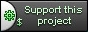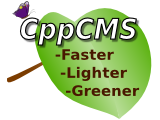# Difference "cppcms::base_view & cppcms::base_content" ver. 5 versus ver. 6

## Content:

 `This content is down.` ``` ``` ```## cppcms::base\_content ``` ``` ``` ```This is a simple polymorphic class from which every cppcms content template should be derided. It allows dynamic casting of the content for the target application: ``` ``` ``` ``` class base_content { ``` ``` public: ``` ``` virtual ~base_content() {}; ``` ``` }; ``` ``` ``` ```## cppcms::base\_view ``` ``` ``` ```### Members ``` ``` ``` ```- **`worker_thread &worker`** -- reference to the worker thread that called the rendering procedure. ``` ```- **`ostream &cout`** -- reference to the output stream that output is rendered to. ``` ``` ``` ```### How Derived Classes are created: ``` ``` ``` ```This is the class that every view generated from the template system is derived from. ``` ``` ``` ```Every derived class defines the important public member --- `content` --- the reference to the user defined content class. ``` ``` ``` ```It also creates member functions for each implemented template. For example: ``` ``` ``` ``` <% c++ #include "data.h" %> ``` ``` <% namespace my_view %> ``` ``` <% class message uses data::message %> ``` ``` <% template render() %> ``` ``` ``` ``` ``` ```

<% message %> World!

``` ``` ``` ``` ``` ``` <% end template %> ``` ``` <% end class %> ``` ``` <% end namespace %> ``` ``` ``` ```The above template is rendered to C++ output: ``` ``` ``` ``` #include "data.h" ``` ``` namespace my_view { ``` ``` struct message :public cppcms::base_view ``` ``` { ``` ``` data::message &content; ``` ``` cppcms::transtext::trans const *tr; ``` ``` message(cppcms::base_view::settings _s,data::message &_content): ``` ``` cppcms::base_view(_s),content(_content) ``` ``` { ``` ``` tr=_s.worker->domain_gettext("my_view"); ``` ``` }; ``` ``` virtual void render() { ``` ``` cout<<"\n" ``` ``` "\n" ``` ``` " \n" ``` ``` "

"; ``` ``` cout<\n" ``` ``` " \n" ``` ``` "\n" ``` ``` ""; ``` ``` } // end of template render ``` ``` }; // end of class message ``` ``` } // end of namespace my_view ``` ``` ``` ``` ``` ```### Member functions ``` ``` ``` ```#### Default filter ``` ``` ``` ``` template ``` ``` string escape(T const &v) ``` ``` ``` ```This is the default filter. It performs the rendering of the input parameter to text. This function is always called when the template parameter is substituted without filters, i.e.: ``` ``` ``` ``` <% param %> ``` ``` ``` ```is converted to ``` ``` ``` ``` cout< ``` ``` ``` ```- **`string intf(int val,string f)`** -- format integer number. It uses `boost::format` for this purpose. For example: ``` ``` ``` ``` Decimal <% num %> is hexadecimal <% num | intf("%x"); %> ``` ``` ``` ```- **`string strftime(std::tm const &t,string f)`** -- formats the output string using std::strftime call. For example: ``` ``` ``` ``` <% date | strftime("%d/%m/%Y") %> ``` ``` ``` ``` It has tho following "shortcuts" for showing the date only, the time or the time with seconds: ``` ``` ``` ``` string date(std::tm const &t); ``` ``` string time(std::tm const &t); ``` ``` string timesec(std::tm const &t); ``` ``` ``` ```- **`string urlencode(string const &s);`** -- encode string for URL. For example: ``` ``` ``` ``` ``` ``` ``` ```#### Other member functions ``` ``` ``` ``` boost::format format(string const &f); ``` ``` ``` ```Function that returns i\a `boost::format` object constructed with `f` and with disabled exceptions. In generally `boost::format` throws an exception in case of an incorrect format or parameters list --- this is not such a good behavior for displaying various data. ``` ``` ``` ```It is used mostly by gettext and ngettext implementations. For example ``` ``` ``` ``` <% ngt "We have one apple","We have %1% apples",n using n %> ``` ``` ``` ```Is rendered to: ``` ``` ``` ``` cout<gettext("We have one apple","We have %1% apples",n)) % escape(n); ``` ``` ``` ```Thus it would not throw an exception in case of "one apple". ``` ``` ```

## Sidebar:

 `This content is down.` ```## Releated ``` ``` ``` `- [cppcms::application](/wikipp/en/page/ref_cppcms_application)`

## About

CppCMS is a web development framework for performance demanding applications.

## Support This Project